## TN State Board 11th Chemistry Important Questions Chapter 8 Physical and Chemical Equilibrium

Question 1.
Name the two phases in equilibrium when ice cubes along with thermos flask at 0°C and 1 atm pressure.
H2O (s) ⇌ H2O (l) at 0°C and 1 atm.

Question 2.
HO (l) and H2O (v) are in equilibrium with each other. At what temperature and pressure, they exist in equilibrium?
H2O (l) ⇌ H2O (V) exist in equation at 373K and 1 atm pressure.
(Generally L ⇌ V equilibrium exist at the boiling point of the liquid at 1 atm pressure).Question 3.
I2 (s) ^ I2 (v). What type of equilibrium it is?
It is solid – vapour equilibrium that exists at the sublimation temperature and at 1 atm pressure.

Question 4.
Give exarnpies for reactions in which
(i) kp = Kc
(ii) Kp > Kc
(iii) Kp < Kc.
Kp = Kc × (RT)∆n
Where,
∆n = (No. of mol of gaseous products) – (No. of mol of gaseous reactants)

(i) Kp = Kc; ∆n = 0
eg: H2 (g) + I2 (g) ⇌ 2HI(g)
Np (g) + Op (g) ⇌ 2NO (g)
[In general, if the number of mol of gaseous products and reactants are the same, ∆n = 0, i.e., Kp = Kc].

(ii) When the total number of mol of gaseous products is greater than the total number of mol of gaseous reactants, ∆n is +ve. Hence Kp > Kc.
eg: 2NH3 (g) ⇌ N2 (g) + 3H2
PCl5 (g) ⇌ PCl3 (g) + Cl2 (g)

(iii) When the total number of mol. of gaseous products is lesser than the total number of gaseous reactants, An is -ve. i.e., Kp < Kc.
eg: 2H2O (g) + O2 (g) ⇌ 2 H2O(g)
2 SO2 (g) + O2 (g) ⇌ 2 SO2 (g)Question 5.
For. the reactions, N2 + 3H2 ⇌ 2 NH3 and $$\frac{1}{2}$$ N2 + $$\frac{3}{2}$$ N2 ⇌ NH3, Write down the express re a constant K1 and K2. How is K1 related to K2?
For the equation N2 + 3H2 ⇌ 2 NH3

K1 = $$\frac{\left[\mathrm{NH}_{3}\right]^{2}}{\left[\mathrm{~N}_{2}\right]\left[\mathrm{H}_{2}\right]^{3}}$$ ………………(1)

and for the equation,
$$\frac{1}{2}$$ N2 + $$\frac{3}{2}$$ N2 ⇌ NH3

K2 = $$\frac{\left[\mathrm{NH}_{3}\right]}{\left[\mathrm{N}_{2}\right]^{1 / 2}\left[\mathrm{H}_{2}\right]^{3 / 2}}$$ …………(2)

Squaring equation (2)

(K2)2 = $$\frac{\left[\mathrm{NH}_{3}\right]^{2}}{\left[\mathrm{~N}_{2}\right]\left[\mathrm{H}_{2}\right]^{3}}$$

Equations (1) and (3) are the same.
Hence, K1 = (K2)2 or
K2 = $$\sqrt{\mathrm{K}_{1}}$$Question 6.
The equilibrium constant for the reaction N2 + O2 ⇌ 2 NO2 at a particular temperature is 100. Write down the equilibrium constant expressions and determine the values of equilibrium constants for the following reactions.
(a) 2NO2 ⇌ N2 + 2 O2
(b) NO2 ⇌ N2 + O2
The equilibrium constant for the reactions
N2 + O2 ⇌ 2 NO2 is

K1 = $$\frac{\left[\mathrm{NO}_{2}\right]^{2}}{\left[\mathrm{~N}_{2}\right]\left[\mathrm{O}_{2}\right]}$$ = 100 ……….(1)

The equilibrium constant for reaction (a) is
K2 = $$\frac{\left[\mathrm{N}_{2}\right]\left[\mathrm{O}_{2}\right]^{2}}{\left[\mathrm{NO}_{2}\right]^{2}}$$ ………..(2)

This is reciprocal of K1

K2 = $$\frac{1}{K_{1}}=\frac{1}{100}$$ = 1 × 10-2
The equilibrium constant for reaction (b) is [N2] + [O2]

K3 = $$\frac{\left[\mathrm{N}_{2}\right]^{\frac{1}{2}}\left[\mathrm{O}_{2}\right]}{\left[\mathrm{NO}_{2}\right]}$$

Comparing (2) and (3)
K3 = $$\sqrt{K_{2}}=\sqrt{10^{-2}}$$
= 10-1 = 0.1Question 7.
At 700 K, the equilibrium constant Kp for the reaction, 2SO3(g) 2SO2 (g) + O2 (g) is 1.80 × 10-3 KPa. What is the value for Kc for the reaction at the same temperature?
Kp = Kc × (RT)∆n.
given, Kp = 1.80 × 10-3 KPa = 1.80 Pa
1.80 = Kc × (8.314 × 700)1
Kc = $$\frac{1.80}{8.314 \times 700}$$

8.314 × 700 = 3.09 × 10-4 mol m3
= 3.09 × 10-7 mol L

Question 8.
For the reactions
(i) PCl5 (g) ⇌ PCl3 (g) + Cl2 (g), the reaction quotient (Q) is greater than Kc.
(ii) H2 (g) + I2 (g) ⇌ 2HI (g), the reaction quotient (Q) is equal to Kf.
(iii) 2NH3(g) ⇌ N2 (g) + 3H2(g), the reaction quotient (Q) is less than Kc.
Predict the direction of the reaction based on the information given.
(i) Q > Kc => The reaction will proceed in the reverse or backward direction.
(ii) Q = Kc => The reaction is at equilibrium.
(iii) Q < Kc => The reaction will proceed in the forward reaction.Question 9.
Discuss the effect of pressure on the following equilibrium.
(i) N2 (g) + O2 (g) ⇌ 2NO
(ii) PCl3 (g) + Cl2(g) ⇌ PCl5 (g)
(iii)K2 (g) + 3H2 (g) ⇌ 2 NH3 (g)
(i) Since the number of mole of gaseous reactants and products are the same, pressure has no effect on this equilibrium.
(ii) Increase in pressure favour the forward reaction which is accompanied by lesser number of moles.
(iii) The forward reaction is accompanied by decrease in number of moles. Hence increase in pressure favour the formation of NH3.

Question 10.
Discuss the effect of temperature on the following equilibria:
(i) N2 (g); + O2 (g) + heat ⇌ 2NO (g)
(ii) N2 (g) + 3H2 (g) ⇌ 2NH3 (g) 92.2 kJmol-1
(i) Increase in temperature will favour an endothermic reaction. Hence, forward reaction is favoured.
(ii) The forward reaction is exothermic and the reverse reaction is endothermic. According to Le Chatelier’s principle, increase in temperature will favour the . reverse reaction i.e., decomposition of ammonia.

Question 11.
For the reaction, N2 (g) + 3 H2 (g) ⇌ 2NH3 (g), the partial pressures of N2 and H2 are 0.80 and 0.40 atmosphere respectively at equilibrium. The total pressure of the system is 2.80 atmospheres. What is Kp for the reaction?
N2 (g) + 3H2 (g) ⇌ 2 NH3 (g)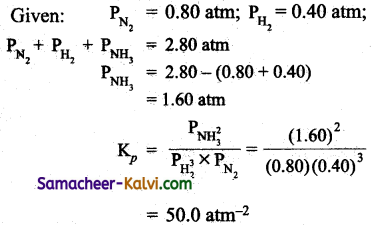Question 12.
The value of Kc for the reaction, 2 A ⇌ B + C is 2.0 × 10-3. At a given time, the composition of the reaction mixture [A] = [B] = [C] = 3 × 10-4 M. In which direction, the reaction will proceed?
For a given reaction,
Q = $$\frac{[\mathrm{B}][\mathrm{C}]}{[\mathrm{A}]^{2}}$$

= $$\frac{\left(3 \times 10^{-4}\right)\left(3 \times 10^{-4}\right)}{\left(3 \times 10^{-4}\right)^{2}}$$ = 1

Q > Kc. Hence the reaction will proceed in backward reaction.

Question 13.
AB2 dissociates AB2 ⇌ AB (g) + B (g). If the initial pressure is 500 mm of Hg and the total pressure at equilibrium is 700 mm of Hg, Calculate Kp for the reaction.
AB2 ⇌ AB (g) + B (g)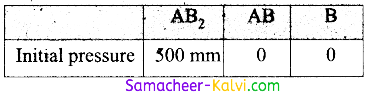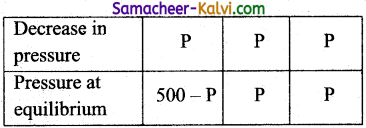Total pressure at equilibrium = 500 – P + P + P = 500 + P
Given 500 + P = 700 (or) P = 200 mm
PAB2 = 500 – 200 = 300 mm
PAB (at equilibrium) = 200 mm
PB (at equilibrium) = 200 mm

Kp = (PAB × PB) / PAB2

= $$\frac{200 \times 200}{300}$$ = 133.3 mmQuestion 14.
The following chemical reaction has attained equilibrium. CO (g) + 2H2 (g) ⇌ CH3OH (g), ∆H = – 92.0 kJ mol-1 What will happen if
(i) The volume of the reaction is suddenly reduced to half?
(ii) The partial pressure of hydrogen is suddenly doubled?
(iii) An inert gas is added to the system?
Kc = $$\frac{\left[\mathrm{CH}_{3} \mathrm{OH}\right]}{[\mathrm{CO}]\left[\mathrm{H}_{2}\right]^{2}}$$;

Kp = $$\frac{{ }^{\mathrm{P}} \mathrm{CH}_{3} \mathrm{OH}}{\mathrm{P}_{\mathrm{CO}} \times \mathrm{P}_{\mathrm{H}_{2}^{2}}}$$
(i) When the volume of the gas is reduced to half, the concentration of each reactant or product becomes double. Then,

Q = $$\frac{2\left[\mathrm{CH}_{3} \mathrm{OH}\right]}{2[\mathrm{CO}] \times 2\left[\mathrm{H}_{2}\right]^{2}}$$

= $$\frac{1}{4}$$ Kc

As Q < Kc, the equilibrium will shift in the forward reaction i.e., more CH3OH will be formed to make Q = Kc

(ii) Q = $$\frac{2 \mathrm{P}_{\mathrm{CH}_{3} \mathrm{OH}}}{2 \mathrm{P}_{\mathrm{CO}} \times 2 \mathrm{P}_{\mathrm{H}_{2}^{2}}}=\frac{1}{4} \mathrm{~K}_{P}$$

As Q < Kp equilibrium-will shift in the forward direction to make Q = Kp.

(iii) As volume remains constant, molar concentrations will not change. Hence, no effect on equilibrium.

Question 15.
SO2Cl2 + heat ⇌ SO2 + Cl2
What will happen to the temperature of the system, if some Cl2 is added into it, at constant volume? Give reason.
In adding chlorine, number of mole of chlorine increases. Hence to reduce the number of mol, the reverse reaction takes place. As the reverse reaction is exothermic, the temperature of the system will increase.Question 16.
Why does ice melts slowly at higher altitudes? Melting of ice is an endothermic process accompanied by decrease in volume. At higher altitudes, pressure as well as temperature is low. In the equilibrium, ice ^ Water, both factors follow the reverse process. Hence, melting is slow.

Question 17.
2 N2O (g) + O2 (g) ⇌ 4 NO (g); ∆H > 0. What is the effect on equilibrium when
(i) Volume of the vessel increases?
(ii) Temperature decreases?
(i) For the given reaction,
K = $$\frac{[\mathrm{NO}]^{4}}{\left[\mathrm{~N}_{2} \mathrm{O}\right]^{2}\left[\mathrm{O}_{2}\right]}$$

When the volume of the vessel increases, number of mol per unit volume (molar concentration) of each reactant and product decreases. As there are 4 concentration terms in the numerator but 3 concentration terms in the denominator, to keep Kp constant, the decrease in [N2O] and [O2] should be more i.e., the equilibrium will shift in the forward reactions.

Alternatively, increase in volume of the vessel, means decrease in pressure. As forward reaction is accompanied by increase in number of moles (P ∝ n), decrease in pressure will favour the forward reaction, (Le Chatelier principle).

(ii) As ∆H is +ve, the reaction is endothermic i.e., decrease in temperature will favour the direction in which heat is absorbed, i.e., backward reaction.Question 18.
Discuss the effect of temperature and pressure on the equilibrium.
2 SO2 (g) + O2 (g) ⇌ 2 SO3 (g) +193.2 kJ.
(i) Effect of temperature:
Increase in temperature favour the endothermic reaction i.e., backward reaction. The formation of SO3 is favoured at low temperature. An optimum temperature of 673 – 723 K is used to get the maximum yield of SO3.

(ii) Effect of pressure:
Increase in pressure favour the reaction accompanied by lesser number of moles. Hence increase in pressure faivour the forward reaction.

Question 19.
Based on the Le Chatelier principle, mention the conditions under which the maximum yield of the products in the following:
(i) Manufacture of NH3
N2 (g) + 3H2 (g) ⇌ 2NH3 (g);
∆H = -92 kL mol-1

(ii) Manufacture of NO
N2 (g) + O2 (g) ⇌ 2NO(g);
∆H = 180.7 kJ mol-1

(iii) Manufacture of SO3
2SO2(s) + O2 (g) ⇌ 2SO3 (g);
∆H = -193.2 kJ mol-1
(i) It is an exothermic reaction, and, accompanied by decrease in number of moles. Hence, it is favoured by low temperature and high pressure.
(ii) It is endothermic reaction favoured by high temperature. Pressure has no effect. .
(iii) Same as (I).Question 1.
For the reaction, PCl3 (g) + Cl2 (g) ⇌ PCl5 (g), the value of Kc at 250°C is 26. The value of Kp at this temperature will be:
(a) 0.61
(b) 0.57
(c) 0.83
(d) 0.46
(a) 0.61
Hint:
Kp = Kc × (RT)∆n
= 26 × (0.082 × 523)-1 = 0.61

Question 2.
The Kp / Kc for the reaction,
CO (g) + O2 (g) ⇌ CO(g) is:
(a) 1
(b) RT
(c) RT $$-\frac{1}{2}$$
(d) (RT)$$\frac{1}{2}$$
(c) RT $$-\frac{1}{2}$$
Hint:
$$\frac{\mathrm{K}_{p}}{\mathrm{~K}_{c}}$$ = (RT)∆n
= (RT)$$-\frac{1}{2}$$Question 3.
For the reaction, N2 (g) + O2 (g) ⇌ 2NO (g), the value of Kc at 800°C is 0.1. When the equilibrium concentrations of both the reactants is 0.5 mol, what is the value of Kp at this temperature?
(a) 0.5
(b) 0.1
(c) 0.01
(d) 0.025
(b) 0.1
Hint:
∆n = 0; Kp = Kc

Question 4.
In a reversible reaction, two substances are in equilibrium. If the concentration of each reactant is doubled, the equilibrium constant will be:
(a) reduced to half its original value
(b) reduced to one fourth of its original value
(c) doubled
(d) constant
(d) constant
Hint:
‘K’ is the ratio between the product of concentration of the products to the product of the concentration of the reactants. If both the numerator and denominator are doubled, K will remain constant.Question 5.
The equilibrium constant for the reaction,
N2 (g) + O2 (g) ⇌ 2NO(g) is 4 × 10-4 at 2000K.
In the presence of the catalyst, the equilibrium is attained ten times faster. Therefore the equilibrium constant in the presence of a catalyst is:
(a) 40 × 10-4
(b) 4 × 10-4
(c) 4 × 10-3
(d) difficult to compute without more data.
Ans :
(b) 4 × 10-4
Hint:
A catalyst helps to attain equilibrium quickly. It does not change the value of equilibrium constant.

Question 6.
Le Chatelier’s principle is not applicable to:
(a) Fe (s) + S (s) ⇌ FeS (s)
(b) H2 (g) + I2 (g) ⇌ 2 SO3 (g)
(c) N2 (g) + 3H2 (g) ⇌ 2NH3 (g)
(d) N2(g) + P2 (g) ⇌ 2NO (g)
Ans :
(a) Fe (s) + S (s) ⇌ FeS (s)
Hint:
The increase in temperature or pressure does not have any impact on solids;

Question 7.
In which of the following reactions, the yield of the products decreases by increasing the pressure?
(a) 2SO2 (g) + O2 (g) ⇌ 2SO3 (g)
(b) K2 (g) + 3 H2 (g) ⇌ 2NH3 (g)
(c) PCl5 (g) ⇌ PCl3 (g) + Cl2 (g)
(d) N2 (g) + O2 (g) ⇌ 2NO(g)
(c) PCl5 (g) ⇌ PCl3 (g) + Cl2 (g)
Hint:
Increasing the pressure towards the reverse reaction, hence the yield of the product [OR] Number of mol of gaseous products > Number of mol of gaseous reactants.Question 8.
Formation of SO3 takes place according to the reaction
2 SO2 + O2 2 SO3
∆H = – 45.2 kcal. Which of the following favours the formation of SO3?
(a) Increase in temperature
(b) Increase in pressure
(c) Removal of oxygen
(d) Increase in volume
(b) Increase in pressure
Hint:
Increase in pressure favour the reaction accompanied by decrease in number of moles of the gas.

Question 9.
For a given exothermic reaction Kp and Kp‘ are the equilibrium constants at temperatures T1 and T2. Assuming the heat of reaction is constant in the temperature range between T1 and T2, which of the following relationship holds good?
(a) Kp > Kp
(b) Kp < Kp
(c) Kp = Kp
(d) Kp = $$\frac{1}{K_{b}}^{\prime}$$
(a) Kp > Kp
Hint:
According to van’t Hoff equation
log $$\frac{\mathrm{K}_{p}{ }^{\prime}}{\mathrm{K}_{p}}$$ = $$\frac{\Delta \mathrm{H}}{2.303 \mathrm{R}}\left[\frac{1}{\mathrm{~T}_{2}}-\frac{1}{\mathrm{~T}_{1}}\right]$$

For exothermic reaction, ∆H is negative also T2 > T1

∴ $$\frac{1}{\mathrm{~T}_{2}}-\frac{1}{\mathrm{~T}_{1}}$$ = – ve

∴ log $$\frac{\mathrm{k}_{p}^{\prime}}{\mathrm{K}_{p}}$$ = – ve
(or) log Kp‘ – log Kp = – ve
i.e., log Kp‘ < log Kp or
Kp‘ < Kp or
Kp > KpQuestion 10.
‘a’ moles of PCl5 are heated in a closed container to equilibrate
PCl5 (g) ^ PCl3 (g) + Cl2 (g). at a pressure of ‘P’ atm. If, ‘x’ mole of PCl5 dissociate at equilibrium, then:
(a) $$\frac{x}{a}=\left(\frac{\mathrm{K}_{p}}{\mathrm{P}}\right)^{\frac{1}{2}}$$
(b) $$\frac{x^{\prime}}{a}=\frac{K_{p}}{K_{p}+P}$$
(c) $$\frac{x}{a}=\left(\frac{\mathrm{K}_{p}}{\mathrm{~K}_{p}+\mathrm{P}}\right)^{\frac{1}{2}}$$

(d) $$\frac{x}{a}=\left(\frac{K_{p}+P}{K_{p}}\right)^{1 / 2}$$

(c) $$\frac{x}{a}=\left(\frac{\mathrm{K}_{p}}{\mathrm{~K}_{p}+\mathrm{P}}\right)^{\frac{1}{2}}$$
Hint: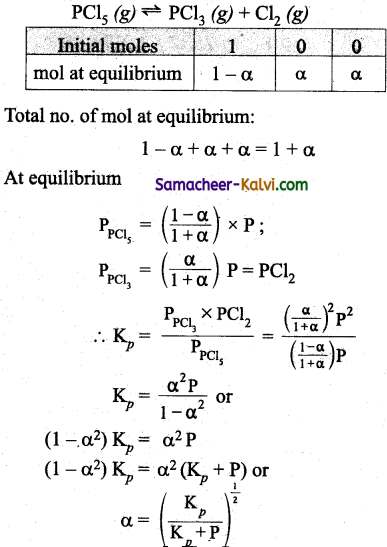Question 11.
If the concentration of OH ions in the reaction Fe(OH)3 ⇌ Fe+3 (aq) + 3 OH is decreased by $$\frac{1}{4}$$ times, then the equilibrium concentration of Fe+3 ions will increase by:
(a) 8 times
(b) 16 times
(c) 64 times
(d) 4 times
(c) 64 times
Hint:
K = $$\frac{\left[\mathrm{Fe}^{+3}\right]\left[\mathrm{OH}^{-}\right]^{3}}{\left[\mathrm{Fe}(\mathrm{OH})_{3}\right](s)}$$
= xy3 (aq)

If [OH] is decreased to $$\frac{y}{4}$$ to keep K constant.
x’ ($$\frac{y}{4}$$)3 = xy3 or x’ = 64x

Question 12.
Five mol of SO2 and 5 mol of O2 are allowed to react. It was found that 60% of SO2 is used up. If the pressure of the mixture is one atmosphere, the partial pressure of O2 is:
(a) 0.52 atm
(b) 0.21 atm
(c) 0.41 atm
(c) 0.82 atm
Ans :
(c) 0.41 atm
Hint:
2SO2 (g) + O2 (g) ^ 2SO3 (g)
Initial moles 5 5 0
As 60% of SO2 is used Up, the number of SO2 Used up is $$\frac{60}{100}$$ × 5 = 3.

∴ no. of mol of SO2 in equilibrium: 5 – 3 = 2
As 2 mol of SO2 react with 1 mol of O2 = $$\frac{1}{2}$$
3 mol of SO2 will react with = $$\frac{1}{2}$$ × 3 = 1.5 mol of O2
∴ number of mol of O2 at equilibrium = 5- 1.5 = 3.5
As 2 mol of SO2 produce 2 mol of SO3, no. of mol of SO3 at equilibrium = 3 mol.
∴ Total no. of mol at equilibrium = 2 + 3.5 + 3 = 8.5
∴ PO2 = $$\frac{x_{o_{2}}}{x_{\text {total }}}$$ × Ptotal
= $$\frac{1}{4}$$ × 1 = 0.41 atmQuestion 13.
9.2 grams of N2O4 (g) is taken in a closed one litre vessel and heated till equilibrium is reached. What is the equilibrium constant (in mol lit-1) (molecular weight of N2O4 = 92)?
(a) 0.1
(b) 0.2
(c) 0.4
(d) 2
(b) 0.2
Hint:
Initial [N2O4] = $$\frac{9.2}{92}$$ mol L-1 = 0.1 mol L-1
At equilibrium (after 50% dissociation)
[N2O4] = 0.05 ML;
[NO2] = 0.1 M
K = $$\frac{\left[\mathrm{NO}_{2}\right]^{2}}{\left[\mathrm{~N}_{2} \mathrm{O}_{4}\right]}=\frac{(0.1)^{2}}{0.05}$$ = 0.2

Question 14.
One, mole of water and one mole of carbon monoxide are taken in a 10 litre flask and heated to 725K. At equilibrium 40% of water (by mass) reacts with CO according to the equation.
H2O (g) + CO (g) – H2 (g) + CO2 (g)
The equilibrium constant for the reaction is:
(a) 0.444
(b) 0.044
(c) 4.44
(d) 444
(a) 0.444
Hint:
At equilibrium,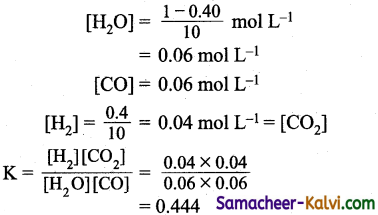Question 15.
A sample of HI(g) is placed in a flask at a pressure of 0.2 atm. At equilibrium, the partial pressure of HI (g) is 0.04 atm. The Kp for the equilibrium is:
(a) 4
(b) 0.4
(c) 40
(c) 0.04
(a) 4
Hint: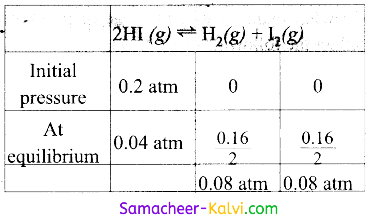Decrease in pressure of HI = 0.2 – 0.04 = 0.16 atm
Kp = $$\frac{{P_{H_{2}}} \times P_{I_{2}}}{P_{H I}^{2}}$$

= $$\frac{0.08 \mathrm{~atm} \times 0.08 \mathrm{~atm}}{10.04 \mathrm{~atm}^{2}}$$ = 4.0Question 16.
For the hypothetical reaction, the equilibrium constant (K) values are given:
A ⇌ B; K1 = 2.0
B ⇌ C; K1 =4.0
C ⇌ D; K1 = 3.0
The equilibrium constant K4 for the reaction, A = D is:
(a) 48
(b) 6
(c) 12
(d) 24
Ans :
(d) 24
Hint:
K4 = K1 × K2 × K3 = 2 × 4 × 3 = 24

Question 17.
Kp for the following reaction will be equal to:
3 Fe(s) + 4 H2O (g) Fe3O4 (s) + 4 H2 (g) is:
(a) $$\frac{P_{H_{2}}^{4}}{P_{H_{2} O}}$$

(b) $$\frac{P_{H_{2}}^{4}}{\left(P_{H_{2}} O\right)^{4}}$$

(c) $$\mathrm{P}_{\mathrm{H}_{2}}^{4} \times \mathrm{P}_{\mathrm{Fe}_{3} \mathrm{O}_{4}}$$

(d) $$\mathrm{P}_{\mathrm{H}_{2}} \times \mathrm{P}_{\mathrm{Fe}_{3} \mathrm{O}_{4}} / \mathrm{P}_{\mathrm{Fe}}$$
(b) $$\frac{P_{H_{2}}^{4}}{\left(P_{H_{2}} O\right)^{4}}$$
Hint:
For Kp only partial pressure of gaseous substances are taken. The active mass of solids are taken as unity.Question 18.
In what manner, will increase of pressure affect the following equilibrium?
C (s) + H2(g) ⇌ CO (g) + H (g)
(a) Shift in the forward reaction.
(b) Shift in the reverse reaction.
(c) Increase in the yield of hydrogen.
(d) No effect.
(b) Shift in the reverse reaction.
Hint:
Increase in pressure, will favour the reaction which is accompanied by decrease in number of moles of gases.

Question 19.
What would happen to a reversible dissociation reaction at equilibrium when an inert gas is added while the pressure remains unchanged?
(a) Less of the product will be formed.
(b) More of the product will be formed.
(c) More of the reactants will be formed.
(d) It remains unaffected.
(b) More of the product will be formed.
Hint:
A dissociation reaction involves the formation of more products.
A ⇌ B + C

At constant pressure, volume is proportional to number of moles. Addition of inert gas increases the number of mole (or) volume of the system. On account of this, the number of moles per unit volume (molar concentration) of the reactant and products will decrease. In order to overcome this stress, the equilibrium will shift in the direction in which there is an increase in the number of moles of the gases.Question 20.
Choose the odd man out of the equilibrium that is not influenced by pressure.
(a) N2 (g) + 3 H2 (g) ⇌ 2 NH3 (g)
(b) CO2 (g) + 3H2 (g) ⇌ CH4 (g) + H2O (g)
(c) PCl5 (g) ⇌ PCl3 (g) + Cl2 (g)
(d) 2 HI (g) ⇌ H2 (g) + I2 (g)
(d) 2 HI (g) ⇌ H2 (g) + I2 (g)

Question 21.
Select the odd man out of the following reactions Kp equal to Kc?
(a) H2 (g) + I2 (g) ⇌ 2HI(g)
(b) 2NO2 (g) ⇌ N2O4 (g)
(c) PCl5 (g) ⇌ PCl3 (g) + Cl2 (g)
(d) SOCl2 (g) ⇌ SO2 (g) + Cl2 (g)
(a) H2 (g) + I2 (g) ⇌ 2 HI(g)

Question 22.
The correct pair of substances whose molar concentration is taken as unit counting in the expression for equilibrium constant.
(a) a 0.1M solution of CH3COOH and a 0.1 M Solution of ethanol.
(b) 0.5 mol of nitrogen and 1.0 mol of oxygen in equilibrium with nitro oxide
(c) Solid calcium carbonate and solid CaO in the equilibrium CaCO3 ⇌ CaO + CO2
(d) CH3COOC2H2 (l) and H2O (l) in equilibrium with CH3COOH and C2H5O4
(c) Solid calcium carbonate and solid CaO in the equilibrium CaCO3 ⇌ CaO + CO2Question 23:
Assertion (A):
The equilibrium constant is fixed and characteristic for any given chemical reaction at a specified temperature.
Reason (R):
The composition of the final equilibrium mixture at a particular temperature depends upon the starting amount of reactants.
(a) If both assertion (A) and reason (R) are true and reason is the correct explanation of assertion.
(b) If both assertion (A) and reason (R) are true, but reason is not the correct explanation of assertion.
(c) If assertion is true but reason is false.
(d) If both assertion and reason are false.
(a) If both assertion (A) and reason (R) are true and reason is the correct explanation of assertion.

Question 24.
Assertion (A):
Equilibrium constant of a reaction increases, if the temperature is raised.
Reason (R):
The forward reaction becomes faster as the temperature is raised.
(a) If both assertion (A) and reason (R) are true and reason is the correct explanation of assertion.
(b) If both assertion (A) and reason (R) are true, but reason is not the correct explanation of assertion.
(c) If assertion is true but reason is false.
(d) If both assertion and reason are false.
(c) If assertion is true but reason is false.

Question 25.
Assertion (A):
If the standard free energy change of a reaction is zero, this implies that equilibrium constant of the reaction is unity.
Reason (R):
For a reaction in equilibrium, equilibrium constant is always unity.
(a) If both assertion (A) and reason (R) are true and reason is the correct explanation of assertion.
(b) If both assertion (A) trad reason (R) are true, but reason is not the correct explanation of assertion.
(c) If assertion is true but reason is false.
(d) If both assertion and reason are false.
(c) If assertion is true but reason is false.Question 26.
Assertion (A):
For the reaction
2 NO (g) + O2 (g) HF ⇌ 2NO2 (g), increase in pressure favours the forward reaction.
Reason (R):
The reaction is exothermic.
(a) If both assertion (A) and reason (R) are true and reason is the correct explanation of assertion.
(b) If both assertion (A) and reason (R) are true, but reason is not the correct explanation of assertion.
(c) If assertion is true but reason is false.
(d) If both assertion and reason are false.
(b) If both assertion (A) and reason (R) are true, but reason is not the correct explanation of assertion.

Question 27.
Assertion (A):
Adding an inert gas to dissociation equilibrium of N204 at constant pressure and temperatures increases the dissociation.
Reason (R):
Molar concentration of the reactants and products decrease.
(a) If both assertion (A) and reason (R) are true and reason is the correct explanation of assertion.
(b) If both assertion (A) and reason (R) are true, but reason is not the correct explanation of assertion.
(c) If assertion is true but reason is false.
(d) If both assertion and reason are false.
(a) If both assertion (A) and reason (R) are true and reason is the correct explanation of assertion.Question 28.
The gaseous reaction, SO2 + Cl2 ⇌ SO2Cl is exothermic and reversible. A mixture of SO2 (g), Cl2 (g), and SO2Cl2 (g) is at equilibrium in a closed container. Now, a certain quantity of extra SO2 is introduced into the container, the volume remaining the same. Which of the following is/are true?
(a) The pressure inside the container will not change
(b) The temperature will not change
(c) The temperature will increase
(d) The temperature will decrease.
(c) The temperature will increase
Hint:
SO2 + Cl2 ⇌ SOCl2 + heat.
On adding SO⇌, the equilibrium will shift in the forward reaction, i.e., more heat will be produced so the temperature will increase.

Question 29.
To an equilibrium mixture of
2 SO2 (g) + O2 (g) ⇌ 2SO3 (g)
Some helium, an inert gas is added at constant volume. The addition of helium causes the total pressure to double. Which of the following is true?
(a) The concentration of all the three gases is unchanged.
(b) The concentration of sulphur tri oxide decreases.
(c) The number of moles of sulphur tri oxide increases.
(d) The concentration of sulphur tri oxide increase.
(a) The concentration of all the three gases is unchanged.
Hint:
As the volume remains constant, the concentration of all three gases will remains unchanged an adding helium.Question 30.
The Thermal dissociation of CaCO3 (s) is studied under different conditions.
CaCO3 (s) ⇌ CaO (s) + CO2 (g)
For this the correct statement(s) is (are):
(a) The value of the equilibrium constant Kp = [CaO][CO2]
(b) Removal of CO2 facilitates the formation of CO2
(c) The value of K is dependent on the amount of CaCO3 taken.
(d) Addition of a catalyst, favour the forward reactions.
(b) Removal of CO2 facilitates the formation of CO2

Question 31.
For the reversible reactions
N2 (g) + 3 H2 (g) ⇌ 2NH3 (g) + heat
Choose the correct statement that favours the shifting the equilibrium in the forward reactions.
(a) by increasing the concentration of NH3 (g).
(b) by decreasing the pressure.
(c) by decreasing the concentration of N2 (g) and H2 (g).
(d) by decreasing the temperature and increasing the pressure.
(d) by decreasing the temperature and increasing the pressure.Question 32.
Match the entities in column I with appropriate entities in column II.

 Column I (Process) Column II (Property becoming constant at equilibrium) (i) H2O (s) ⇌ H2O (l) (A) Concentration of the solute at a given temperature. (ii) H2O (l) ⇌ H2O (v) (B) Ratio of concentration of products to reactants at constant temperature. (iii) Sugar (s) ⇌ Sugar solution (C) Melting point at constant and pressure. (iv)CO2 (g) ⇌ CO2 (aq) (D) Vapour pressure at constant temperature.

(a) (i) – (C), (ii) – (D), (iii) – (A), (iv) – (B)
(b) (i) – (D), (ii) – (C), (iii) – (B), (iv) – (A)
(c) (i) – (D), (ii) – (C), (iii) – (A), (iv) – (B)
(d) (i) – (C), (ii) – (A), (iii) – (B), (iv) – (D)
(a) (i) – (C), (ii) – (D), (iii) – (A), (iv) – (B)

Question 33.
Which of the following statements are wrong?
I. Equilibrium constant of a reaction is doubled, of the concentrations of the products become double.
II. If the reaction mixture is compressed to half the volume, equilibrium constant is halved.
III. Equilibrium constant increases with increase in temperature.
IV. Equilibrium concentration increases in the pressure of a catalyst.
(a) I, II, III
(b) II, III, IV
(b) III
(d) all
(c) III
Hint:
The effect of temperature on the equilibrium constant is given by Vant Hoff equation, i.e.,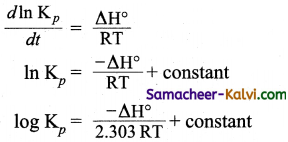Question 34.
Match the entities in column I with appropriate entities in column II.

 Column I Column II (i) Manufacture of NH3 (A) Favoured by high temperature (ii) Manufacture of NO (B) Favoured by increasing temperature and pressure (iii) Melting of ice (C) Equilibrium remains unaffected (iv) Dissociation of PCl5 at constant volume (D) Favoured by low temperature and high pressure

(a) (i) – (D), (ii) – (A), (iii) – (B), (iv) – (C)
(b) (i) – (A), (ii) – (B), (iii) – (D), (iv) – (C)
(c) (i) – (D), (ii) – (C), (iii) – (A), (iv) – (B)
(d) (i) – (B), (ii) – (D), (iii)- (C), (iv) – (A)